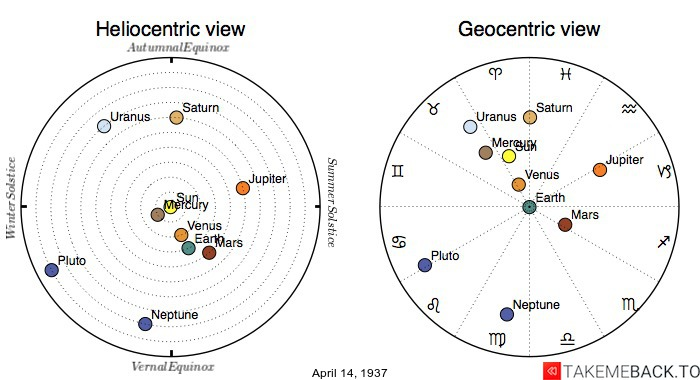## April 14th, 1937 Zodiac and Birth Chart

#### Maps of the planetary positionsNB: The dimensions and the orbits of the planets are not in scale. The zodiac signs in the geocentric view are set assuming that Aries coincides with the vernal equinox and that each of the zodiac signs occupy exactly one-twelfth of the sky.

An observer located in New York City on Wednesday April 14, 1937, would see:Sun The sun rising at 05:18 am and setting at 06:35 pm. The moon, being 21% full, rising at 07:38 am and setting at 11:04 pm. The previous full moon was on Mar 26, 1937 while the next full moon would come on Apr 25, 1937.

The same observer would also see the other Solar System Planets as follows:

astrology_tvMercury Mercury rising at 05:56 am and setting at 08:17 pm with a 52% of surface illuminated. Mercury would be orbiting the Sun at a distance of 0.33 astronomical units (AU) and would be found at a distance of 0.96 astronomical units (AU) from Earth. Given the planet elongation, the observer would see Mercury at an angle of 19° 18' 54.6" with the Sun. The brightness would be -0.29 this day, as measured by the planet magnitude.Venus Venus would rise at 04:55 am and set at 07:04 pm. 0% of Venus would be illuminated by the Sun. Venus distance from the Sun would be 0.72 astronomical units (AU) and its distance from the Earth 0.29 astronomical units (AU). The angular distance in the sky between Venus and the Sun would be 7° 31' 15.0".Mars Mars would look like 95% full, orbiting the Sun at a distance of 1.57 AU and be at 0.66 AU from Earth. The planet would be seen rising at 09:47 pm and setting at 07:25 am. The angular separation of the Sun and the planet, with Earth as the reference point, would be -139° 35' 46.5". The brightness of Mars, that is its magnitude, would be -1.05.Jupiter Jupiter’s distance from the Sun would be 5.19 AU and 5.10 AU from Planet Earth. Jupiter rising and setting times would be 01:29 am and 10:59 am respectively. Jupiter would appear with a 99% illuminated fraction of its surface and a magnitude (brightness of the star) of -2.08. Its angular separation between the Sun would be -89° 19' 33.9".Saturn Saturn would rise at 04:27 am and set at 04:15 pm, appearing 99% full. The angular separation of Saturn from the Sun, as seen by the observer, would be -26° 36' 25.8", its magnitude 1.1. Saturn would orbit the Sun at a distance of 9.57 astronomical units (AU) and would be located at a distance of 10.45 astronomical units (AU) from Earth.Uranus Uranus would appear 99 full, its brightness being 5.87. It would be seen rising at 05:56 am and setting at 07:39 pm. Uranus would be 19.75 AU far from the Sun and 20.73 AU far from Planet Earth (average distance). The observer, looking up into the sky, would see Uranus with a 13° 08' 50.4" angle of separation from the Sun.Neptune Neptune rising at 03:09 pm, and setting at 04:00 am, its magnitude (the degree of brightness of a star) being 7.87. The difference between the celestial longitude of the Sun and that of Neptune would be 141° 38' 08.0". At 30.21 AU distance from the Sun and at 29.41 AU from Earth, 99 % of Neptune would be illuminated by the Sun.Pluto Pluto would be seen as 99% full and would have an elongation of 91° 36' 08.8". It would orbit the Sun at an average distance of 39.56 astronomical units (AU) and be located at an average distance of 39.52 astronomical units (AU) from Earth. The observer would see Pluto rising at 10:47 am and setting at 01:51 am, its magnitude (brightness of the star) being 15.02.

For the same New York observer looking up in the clear night sky around 10 pm of the same day, the solar system planets would be found in the following constellations and at the following coordinates:

PlanetConstellationRight AscensionDeclination
MercuryAries2:46:24.7018:34:44.6
VenusPisces1:37:17.8517:23:21.3